ABSOLUTE STABILITY

So far we have considered the behaviour of numerical methods in the limit as the stepsize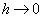. However in practice we must deal with finite stepsizes. To illustrate the problems that might arise consider the mid-point method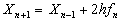This is a linear two-step method. In standard form the method is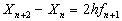thus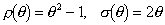Checking consistency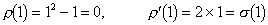The roots of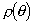are given by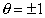hence the method is both consistent and zero-stable and hence convergent.

Now consider the solution of the initial value problem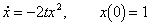by the mid-point method using a stepsize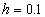. Using Maple we obtain the plot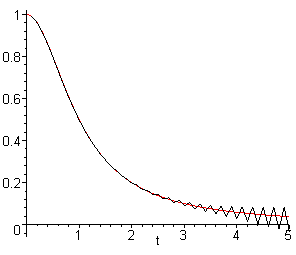Notice that the numerical solution becomes increasingly innacurate, oscillating about the exact solution, as t increases. This behaviour arises because the behaviour of the numerical solution does not mimic that of the exact solution. In this case the problem arises because of a spurious solution of the difference equation corresponding to the root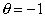of. However the problem can also arise in one-step methods which have no spurious solutions.

The polynomial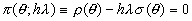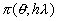is called the stability polynomial of the method. One of the roots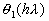will correspond to the true solution, the other roots will lead to spurious solutions whose magnitude will have to be controlled to obtain stability.

Definition  Absolute Stability

A numerical method is said to be absolutely stable for a given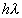if all the roots oflie within the unit circle.

A region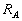of the complex plane is said to be a region of absolute stability if the method is stable for allin.

• Worked Example 7
Find and sketch the region of absolute stability for

(a) Euler's method,

(b) Trapezoidal method.

(a) For Euler's method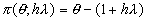Thus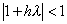is shown below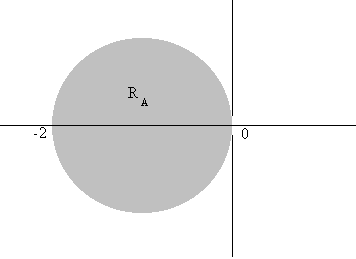(b) For the Trapezoidal method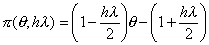Thus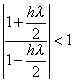giving the regionshown below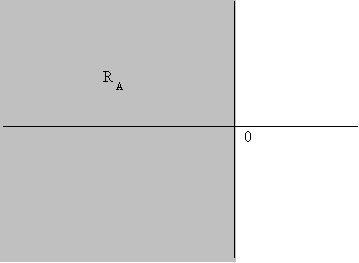For Runge-Kutta methods the stability polynomial has the form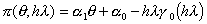where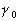is a polynomial for an explicit method and a rational function for an implicit method.

• Worked Example 8
Find and sketch the absolute stability region for the second order Runge-Kutta method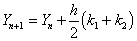where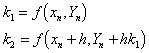The stability polynomial is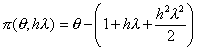For absolute stability we require that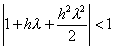In order to draw the region of absolute stability consider the boundary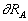of. The locus of this boundary will be the set of complex numbers z such that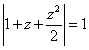Thus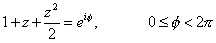In order to obtain the region we need to plot the roots of the quadratic equation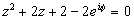for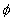in the range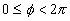. This is best done on a computer. The resulting stability region is shown below: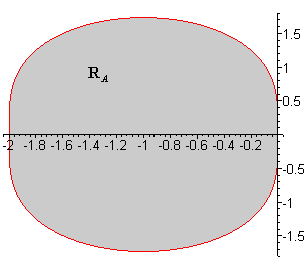The method outlined above is an example of the boundary locus method which is easily implemented for Linear Multistep methods as follows. The stability polynomial isand hence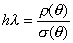but on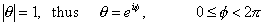Hence the locus of the boundaryis given by the set of complex numbers z satisfying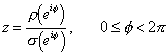• Worked Example 9
Find the region of absolute stability for the Gear method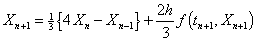The stability polynomial is given by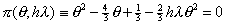Substituting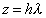and solving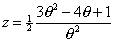Now substitute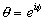to obtain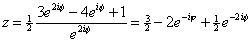which gives the plot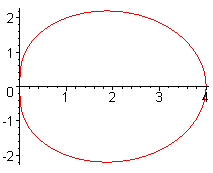In order to determine whetheris the interior or exterior of the closed curve choose a point inside the curve and evaluate the roots of. In this caseconsists of the exterior of the closed curve.

• Worked Example 10
Show that the mid-point method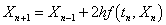has an empty region of absolute stability.

From aboveThus the stability polynomial is given by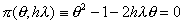Substitutingand solving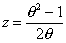Now substituteto obtain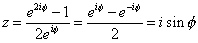which does not bound any region of the complex plane. Henceis empty.